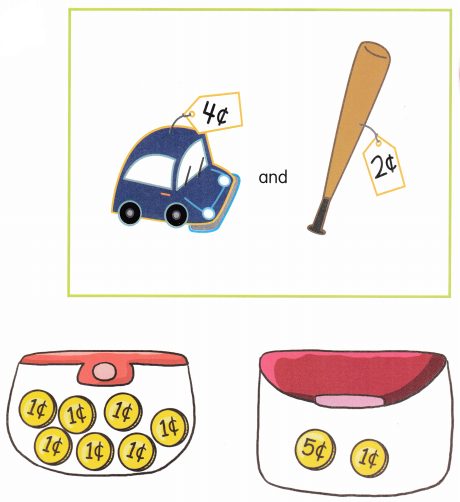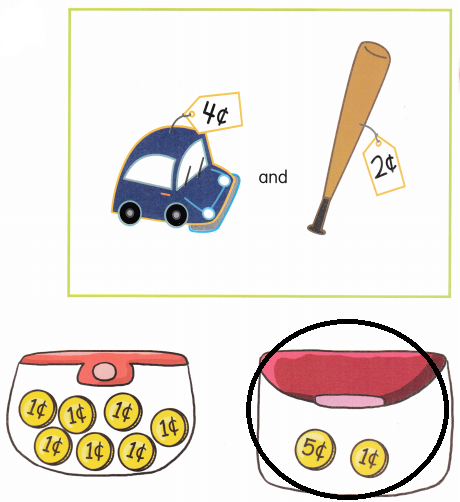# Math in Focus Kindergarten Chapter 20 Answer Key Money

Go through the Math in Focus Grade K Workbook Answer Key Chapter 20 Money to finish your assignments.

## Math in Focus Kindergarten Chapter 20 Answer Key Money

Lesson 1 Coin Values

Match.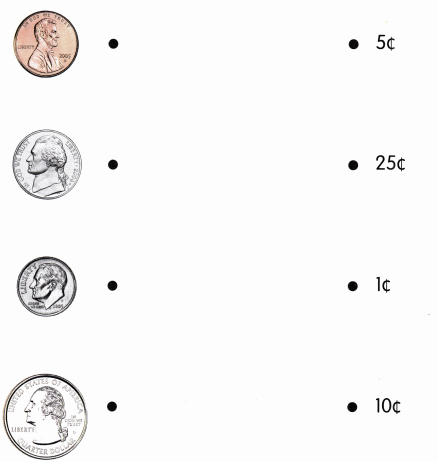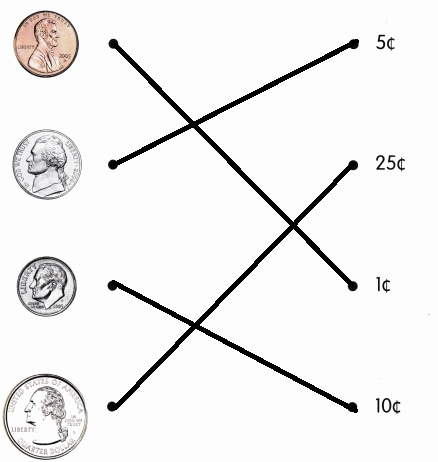Explanation:
1 quarter dollar is equal to 25 cents
1 dime is equal to 10 cents
1 nickel is equal to 5 cents
1 penny is equal to 1 cent

Lesson 2 Counting Coins

How many pennies do you need? Color.

Question 1.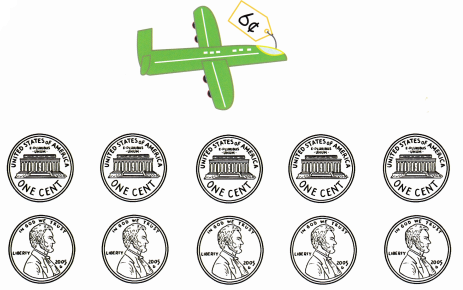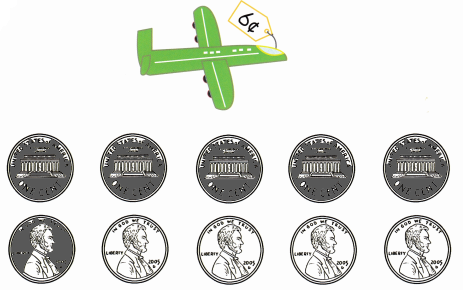Explanation:
The cost of the toy airplane is 6 cents
so, colored 6 cents

Question 2.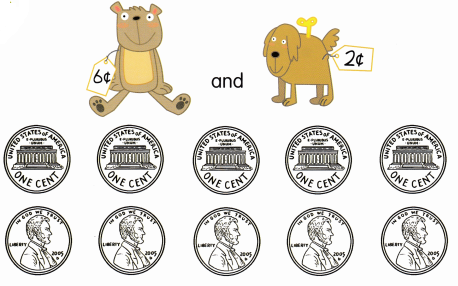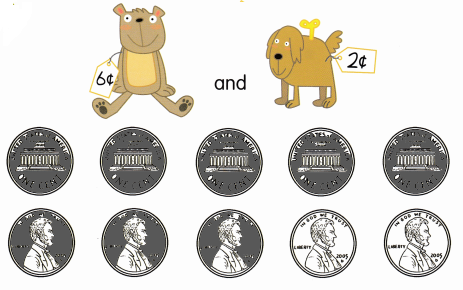Explanation:
The cost of the toys are 6 + 2 = 8
so, colored the 8 cents

Question 3.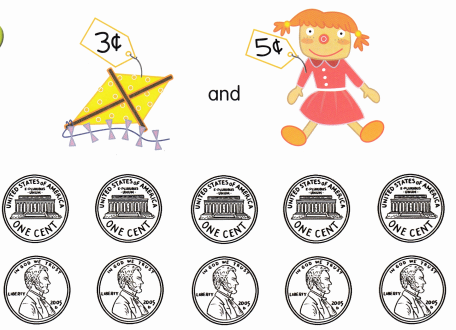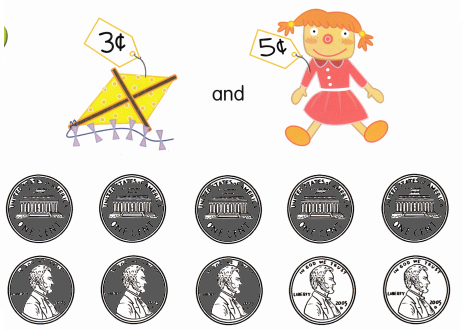Explanation:
The cost of the toys are 3 + 5 = 8 cents
so, colored 8 cents

Question 4.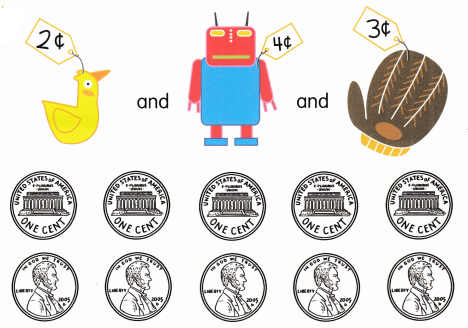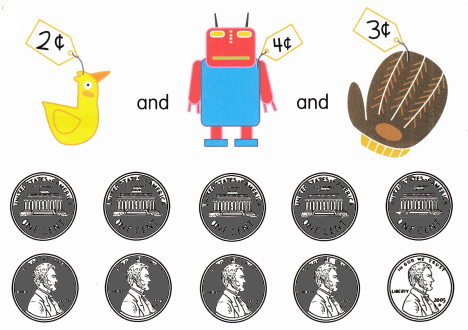Explanation:
The cost of the toys are 2 + 4 + 3 = 9 cents
so, colored 9 cents

How much is needed? Circle the purse.

Question 1.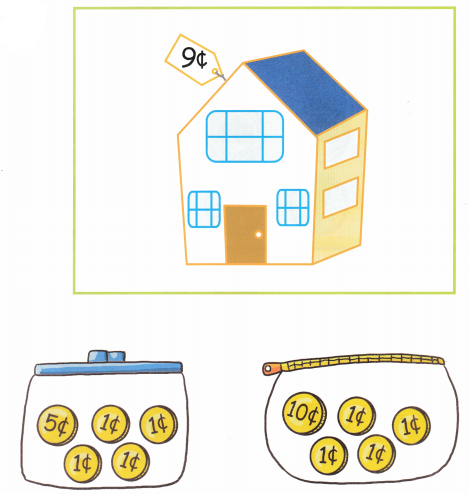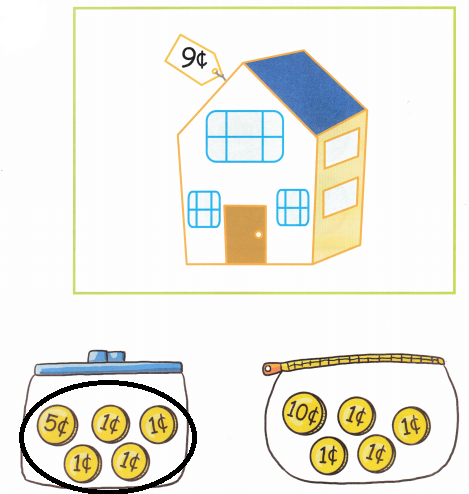Explanation:
The cost of the toy house is 9 cents
5 + 4 = 9 cents
so, colored the first purse

Question 2.